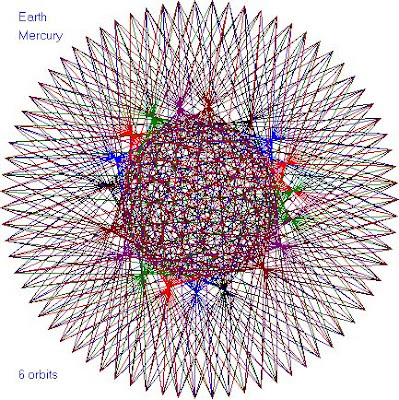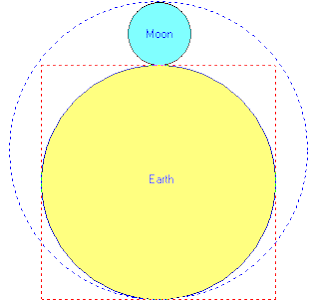## среда, 3 января 2018 г.

### Harmony of the Spheres Dance of the Planets

James Ferguson’s (1710-1776) representation of the apparent

motion of the Sun, Mercury, and Venus from the Earth, based on similar diagrams

by Giovanni Cassini (1625-1712) and  Roger Long (1680-1770). Taken from the “Astronomy” article in the first edition of the Encyclopædia

Britannica (1771; Volume 1, Fig. 2 of Plate XL facing page 449). This

geocentric diagram shows, from the location of the Earth, the Sun’s apparent

annual orbit, the orbit of Mercury for 7 years, and the orbit of Venus for 8

years, after which Venus returns to almost the same apparent position in

relation to the Earth and Sun. In Arabic, Venus is called “El Zahra” – the flower. See HERE + HERE + HERE + HEREEarth – Mercury Cycle. This and all following graphics by John Martineau.Earth – Venus Cycle: Earth = 8 years x 365.256 days/year = 2,922.05 days Venus = 13 years x 224.701 days/year = 2,921.11 days (ie. 99.9%)The radius of the Moon compared to the Earth’s is 3:11

Radius of Moon = 1,080 miles = 3 x 360

Radius of Earth = 3,960 miles = 11 x 360 = 33 x 1 x 2 x 3 x 4 x 5

Radius of Earth plus Radius of Moon = 5,040 miles = 1 x 2 x 3 x 4 x 5 x 6 x 7 = 7 x 8 x 9 x 10

The ratio 3:11 is 27.3%, and the orbit of the Moon takes 27.3 days, which is also the average rotation period of a sunspot. The closest to farthest distance ratio that Venus and Mars each experiences in the Mars-Venus dance is also 3:11. The Earth orbits between them. The sizes of the Moon and the Earth is drawn to scale in the last illustration above, where the perimeters of the dotted square and the dotted circle are of the same length: The perimeter of the dotted red square is 4 x Earth’s diameter = 4 x 7,920 miles = 31,680 miles. The circumference of the dotted blue circle is 2 pi x radius = 2 x 3.142 x 5040 miles = 31,667 miles (ie. 99.9%).

Source Time-Price-Research